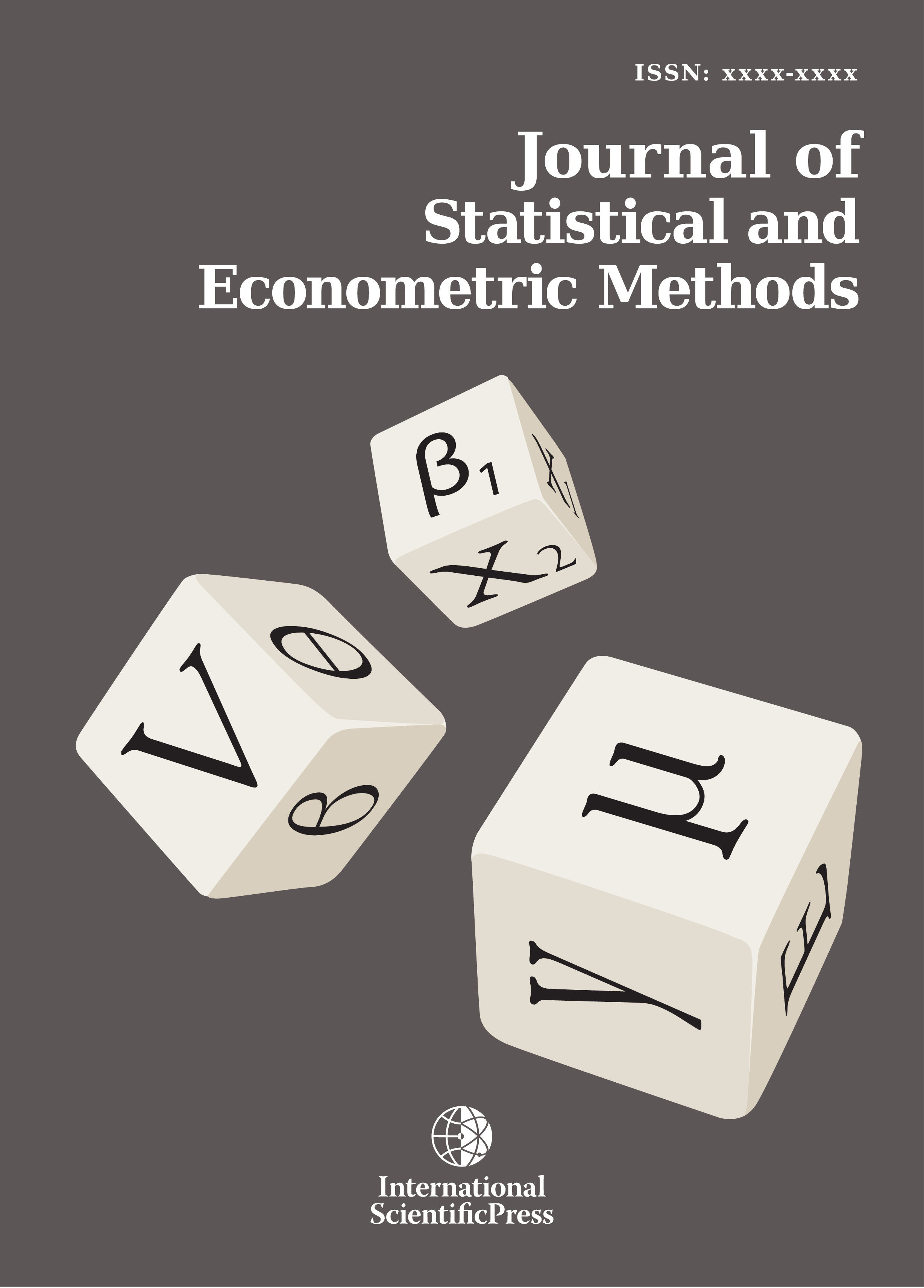# Journal of Statistical and Econometric Methods

#### The Weibull Length Biased Exponential Distribution: Statistical Properties and Applications.

•[ Download ]
• Times downloaded: 421
• Abstract

When the weight function depends on the lengths of the units of interest, the resulting distribution is called length biased. Length biased distribution is thus a special case of the more general form, known as weighted distribution. In this paper, a new three parameter probability distribution called the Weibull Length Biased Exponential distribution is proposed, its statistical properties are studied in minute details. Expansions of the density function of the WLBE Distribution, reliability analysis, asymptotic behavior, moments etc. are discussed in minute details. Maximum likelihood estimation method is employed to determine the estimate of the parameters of the proposed distribution. Simulation studies and application to two life time data is performed to determine the flexibility of the model in modeling lifetime data.

Keywords: Weibull generalized family of distribution, length biased exponential distribution, moments, maximum likelihood, fitted models.ISSN: 2241-0376 (Online)
2241-0384 (Print)